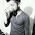## Monday, May 8, 2017

### Flowchart of Simple Interest

#### Simple Interest Calculation of Savings Bank Accounts / Loan Accounts

Following flowchart describes the process of simple interest calculation.

Simple Interest calculation is one of the routine activity performed by Banks. Savings Bank account holder gets interests on their deposits in bank. Loan takers from the bank pay the interest to the bank.

In the following flowchart, P is Principle amount, R is Rate of Interest, N is number of years and I is used for Simple Interest.

Formula to calculate Simple Interest (I) based on given P, R and N is as under:

I  = (P * R * N) / 100

Fig. Flowchart to Calculate Simple Interest

Here, P = Principal amount, R = Rate of Interest, N = No. of years and I = Simple Interest.

More Sample Flowchart

### Flowchart to check positive number. Flowchart to check negative number. Flowchart to check Odd or Even number. Flowchart to display Good morning message based on given time. Flowchart to print A to Z. Flowchart to print 10, 9, ......, 1. Flowchart to Add two numbers. Flowchart to subtract two numbers. Flowchart to print area of square. Flowchart to print area of rectangle. Flowchart to Calculate Simple Interest

1.2.3.